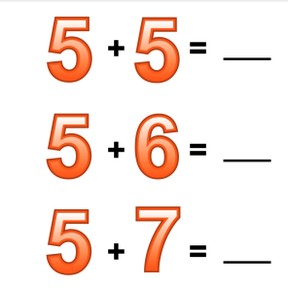Addition to 20 with one more or one less

# Addition to 20 with one more or one less

No account needed.8,000 schools use Gynzy92,000 teachers use Gynzy1,600,000 students use Gynzy

## General

Students learn to add to 20 by counting one more, one less and carrying numbers.

## Relevance

Discuss with students that it is useful to be able to add using one more or one less. If you know when you need to add one more, or one less, you don't need to calculate problems separately.

## Introduction

Ask a student to come to the front and show them a number. The student must take as many steps as shown on the number. The class must count how many steps they take and say the correct number when they stop. Students must then in pairs count backwards from 20 to 10. They must try to count this within the given time.

## Development

Next show the colored pencils and say that there are ten and four are added. Together there are fourteen colored pencils. Discuss that there are other problems you can show with the colored pencils. Ask students what they notice about the problems. Say that there is one less colored pencil every time. There aren't always images that you can count. Sometimes you just see numbers. Compare the numbers. If the first addend is one less than the first addend in the second problem, then the answer is one less too. Show some problems on the interactive whiteboard and have students determine the answers. Ask how they determined their answers. Have students write down the answers to the following problems on a sheet of paper, and have them hold up their papers to check their work.

To check that students are able to add to 20 using one more or one less, you can ask the following questions/give the following exercises:
- What do you look at to see if you are adding one more, or taking one away (one less)?
- Calculate the following: 3+7 and 4+7
- Calculate the following: 10+5, 9+5, and 8+5.

## Guided practice

Students first practice adding with one more or one less with three addition problems. Then they practice adding one more or one less with three and four addition problems.

## Closing

Discuss with students that it is important to be able to add to 20 with one more or one less, so you can quickly solve sets of problems without needing to caclulate each one separately. Show the addition problems on the interactive whiteboard and have students calculate the answer. Ask students how they calculated, and what a useful or clever way to solve might be. Drag the splotches away to show the answers.

## Teaching tips

Students who have difficulty adding with one more or one less can use MAB blocks or other manipulatives. Have them first set out blocks to represent the addition problem. Then have them do a problem with one more. The student must add one block to the blocks they already have. Repeat this to show one less.

## Instruction materials

Optional: MAB blocks or manipulatives

### The online teaching platform for interactive whiteboards and displays in schools

• Save time building lessons

• Manage the classroom more efficiently

• Increase student engagement